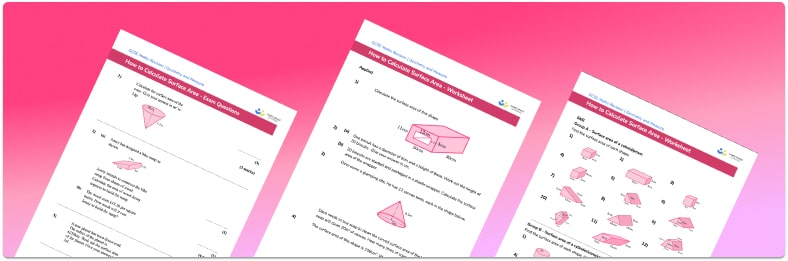# Surface Area Worksheet• Section 1 of the surface area worksheet contains 36 skills-based surface area questions, in 3 groups to support differentiation
• Section 2 contains 4 applied surface area questions with a mix of worded problems and deeper problem solving questions
• Section 3 contains 4 foundation and higher level GCSE exam style surface area questions
• Answers and a mark scheme for all surface area questions are provided
• Questions follow variation theory with plenty of opportunities for students to work independently at their own level
• All questions created by fully qualified expert secondary maths teachers
• Suitable for GCSE maths revision for AQA, OCR and Edexcel exam boards
• There are other printable worksheets with answer keys available, covering surface area of 3D shapes, including surface area of cuboids and surface area of cylinders.

• This field is for validation purposes and should be left unchanged.

You can unsubscribe at any time (each email we send will contain an easy way to unsubscribe). To find out more about how we use your data, see our privacy policy.

### Surface area at a glance

The surface area of a 3D shape is the total area of all of the faces that make up its surface. For example, in order to calculate the surface area of a cube we need to work out the area of one of the square faces of a cube and multiply this by 6 to find the total surface area (because a cube has 6 square faces).

We can also calculate the surface area of more complex prisms (solid shapes with flat faces and constant cross-sections). For example, to find the surface area of cuboids (also known as rectangular prisms, as the cross-section of a cuboid is a rectangle) it is useful to draw its net. Then mark on the dimensions of the cuboid and calculate the area of each rectangular face before summing to find the total surface area. To find the surface area of a triangular prism we use the formula for area of a triangle to calculate the surface area of the two cross-sectional faces, then add together with the area of the three rectangular faces.

There are also formulae for curved surface areas of cones and spheres which are given in GCSE assessments. Students may be asked to work with fractions of a sphere, such as hemispheres or quarter-spheres.

Students are also expected to work out the total surface area of compound shapes which are shapes made from two or more simple 3D shapes. It’s important to think carefully about which faces make up the outside of a compound shape, for example, for a hemisphere stacked on top of a cylinder, one circular face of both the hemisphere and the cylinder will no longer be on the outside surface of the compound shape. Compound shape problems are often posed as word problems relating to real life scenarios.

Care must be taken when working with decimals and surface area of 3D shapes and it is important to only round answers at the end of a calculation.

Looking forward, students can then progress to additional geometry worksheets, for example a volume of prisms worksheet or area of compound shapes worksheet.For more teaching and learning support on Geometry our GCSE maths lessons provide step by step support for all GCSE maths concepts.

## Do you have KS4 students who need more focused attention to succeed at GCSE?There will be students in your class who require individual attention to help them succeed in their maths GCSEs. In a class of 30, it’s not always easy to provide.

Help your students feel confident with exam-style questions and the strategies they’ll need to answer them correctly with our dedicated GCSE maths revision programme.

Lessons are selected to provide support where each student needs it most, and specially-trained GCSE maths tutors adapt the pitch and pace of each lesson. This ensures a personalised revision programme that raises grades and boosts confidence.

Find out more